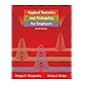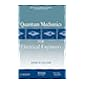Normal view

## Applied Statistics and Probability for Engineers / by Douglas C Montgomery, George C Runger

Material type:TextPublication details: London : John Wiley & sons, 2006cEdition: 4th edDescription: xvi, 768 p. : ill.; 26 cmISBN: 9780471745891 (hbk)Other title: Applied Statistics & Probability for EngineersSubject(s): Probabilities. -- EngineeringDDC classification: 519.5
Contents:
The Role of Statistics in Engineering -- Probability -- Discrete Random Variables and Probability Distributions -- Continuous Random Variables and Probability Distributions -- Joint Probability Distributions -- Random Sampling and Data Description -- Sampling Distributions and Point Estimation of Parameters -- Statistical Intervals for a Single Sample -- Tests of Hypotheses for a Single Sample -- Statistical Inference for Two Samples -- Simple Linear Regression and Correlation -- Multiple Linear Regression -- Design and Analysis of Single-Factor Experiments: The Analysis of Variance -- Design of Experiments with Several Factors -- Nonparametric Statistics -- Statistical Quality Control.
Tags from this library: No tags from this library for this title.Average rating: 0.0 (0 votes)
Item type Current library Call number Status Date due Barcode Item holdsBooks Namal Library
Electrical Engineering
519.5 MON-A 2006 892 (Browse shelf (Opens below)) Available 892
Total holds: 0
##### Browsing Namal Library shelves, Shelving location: Electrical Engineering Close shelf browser (Hides shelf browser)512.7 HOF-I 2011 332 An Introduction to mathematical cryptography 515.64 DIS-C 2012 372 Schaum's outlines control systems 515 VAR-C 2007 2679 Calculus : early transcendentals / 519.5 MON-A 2006 892 Applied Statistics and Probability for Engineers / 529.1 RAM-R 2010 649 Rocket propulsion / 530.12 SUL-Q 2012 9032 Quantum mechanics for electrical engineers 530.141 MIL-F 1998 2954 Foundations of electromagnetic theory /

Index Included

The Role of Statistics in Engineering -- Probability -- Discrete Random Variables and Probability Distributions -- Continuous Random Variables and Probability Distributions -- Joint Probability Distributions -- Random Sampling and Data Description -- Sampling Distributions and Point Estimation of Parameters -- Statistical Intervals for a Single Sample -- Tests of Hypotheses for a Single Sample -- Statistical Inference for Two Samples -- Simple Linear Regression and Correlation -- Multiple Linear Regression -- Design and Analysis of Single-Factor Experiments: The Analysis of Variance -- Design of Experiments with Several Factors -- Nonparametric Statistics -- Statistical Quality Control.

There are no comments on this title.

to post a comment.
Share